# DIGITAL-ANALOG CONVERSION

Connecting digital circuitry to sensor devices is simple if the sensor devices are inherently digital themselves. Switches, relays, and encoders are easily interfaced with gate circuits due to the on/off nature of their signals. However, when analog devices are involved, interfacing becomes much more complex. What is needed is a way to electronically translate analog signals into digital (binary) quantities, and vice versa. An analog-to-digital converter, or ADC, performs the former task while a digital-to-analog converter, or DAC, performs the latter.

An ADC inputs an analog electrical signal such as voltage or current and outputs a binary number. In block diagram form, it can be represented as such: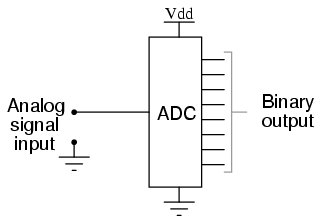A DAC, on the other hand, inputs a binary number and outputs an analog voltage or current signal. In block diagram form, it looks like this: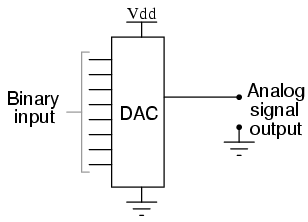Together, they are often used in digital systems to provide complete interface with analog sensors and output devices for control systems such as those used in automotive engine controls: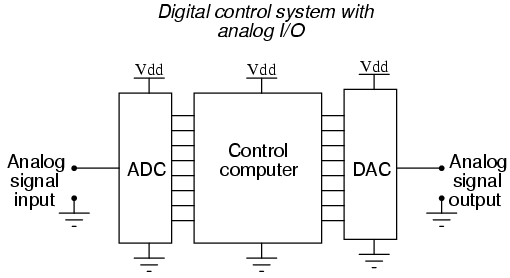It is much easier to convert a digital signal into an analog signal than it is to do the reverse. Therefore, we will begin with DAC circuitry and then move to ADC circuitry.

## The R/2nR DAC

This DAC circuit, otherwise known as the binary-eighted-input DAC, is a variation on the inverting summer op-amp circuit. If you recall, the classic inverting summer circuit is an operational amplifier using negative feedback for controlled gain, with several voltage inputs and one voltage output. The output voltage is the inverted (opposite polarity) sum of all input voltages: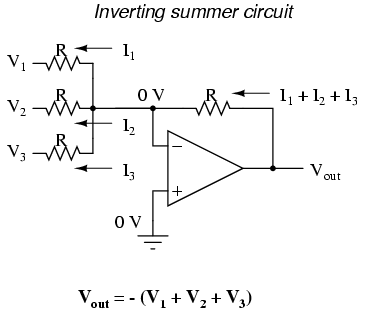For a simple inverting summer circuit, all resistors must be of equal value. If any of the input resistors were different, the input voltages would have different degrees of effect on the output, and the output voltage would not be a true sum. Let’s consider, however, intentionally setting the input resistors at different values. Suppose we were to set the input resistor values at multiple powers of two: R, 2R, and 4R, instead of all the same value R:Starting from V1 and going through V3, this would give each input voltage exactly half the effect on the output as the voltage before it. In other words, input voltage V1 has a 1:1 effect on the output voltage (gain of 1), while input voltage V2 has half that much effect on the output (a gain of 1/2), and V3 half of that (a gain of 1/4). These ratios are were not arbitrarily chosen: they are the same ratios corresponding to place weights in the binary numeration system. If we drive the inputs of this circuit with digital gates so that each input is either 0 volts or full supply voltage, the output voltage will be an analog representation of the binary value of these three bits.If we chart the output voltages for all eight combinations of binary bits (000 through 111) input to this circuit, we will get the following progression of voltages:

———————————
| Binary | Output voltage |
———————————
| 000 | 0.00 V |
———————————
| 001 | -1.25 V |
———————————
| 010 | -2.50 V |
———————————
| 011 | -3.75 V |
———————————
| 100 | -5.00 V |
———————————
| 101 | -6.25 V |
———————————
| 110 | -7.50 V |
———————————
| 111 | -8.75 V |
———————————

Note that with each step in the binary count sequence, there results a 1.25 volt change in the output. This circuit is very easy to simulate using SPICE. In the following simulation, I set up the DAC circuit with a binary input of 110 (note the first node numbers for resistors R1, R2, and R3: a node number of “1” connects it to the positive side of a 5 volt battery, and a node number of “0” connects it to ground). The output voltage appears on node 6 in the simulation: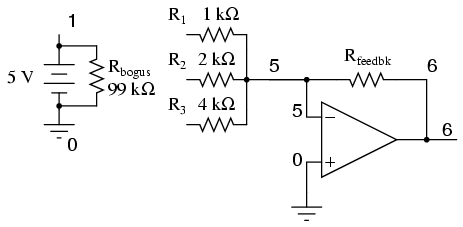binary-weighted dac
v1 1 0 dc 5
rbogus 1 0 99k
r1 1 5 1k
r2 1 5 2k
r3 0 5 4k
rfeedbk 5 6 1k
e1 6 0 5 0 999k
.end

node voltage node voltage node voltage
(1) 5.0000 (5) 0.0000 (6) -7.5000

We can adjust resistors values in this circuit to obtain output voltages directly corresponding to the binary input. For example, by making the feedback resistor 800 Ω instead of 1 kΩ, the DAC will output -1 volt for the binary input 001, -4 volts for the binary input 100, -7 volts for the binary input 111, and so on.

(with feedback resistor set at 800 ohms)
———————————
| Binary | Output voltage |
———————————
| 000 | 0.00 V |
———————————
| 001 | -1.00 V |
———————————
| 010 | -2.00 V |
———————————
| 011 | -3.00V |
———————————
| 100 | -4.00 V |
———————————
| 101 | -5.00 V |
———————————
| 110 | -6.00 V |
———————————
| 111 | -7.00 V |
———————————

If we wish to expand the resolution of this DAC (add more bits to the input), all we need to do is add more input resistors, holding to the same power-of-two sequence of values:It should be noted that all logic gates must output exactly the same voltages when in the “high” state. If one gate is outputting +5.02 volts for a “high” while another is outputting only +4.86 volts, the analog output of the DAC will be adversely affected. Likewise, all “low” voltage levels should be identical between gates, ideally 0.00 volts exactly. It is recommended that CMOS output gates are used, and that input/feedback resistor values are chosen so as to minimize the amount of current each gate has to source or sink.

## The R/2R DAC

An alternative to the binary-weighted-input DAC is the so-called R/2R DAC, which uses fewer unique resistor values. A disadvantage of the former DAC design was its requirement of several different precise input resistor values: one unique value per binary input bit. Manufacture may be simplified if there are fewer different resistor values to purchase, stock, and sort prior to assembly.

Of course, we could take our last DAC circuit and modify it to use a single input resistance value, by connecting multiple resistors together in series: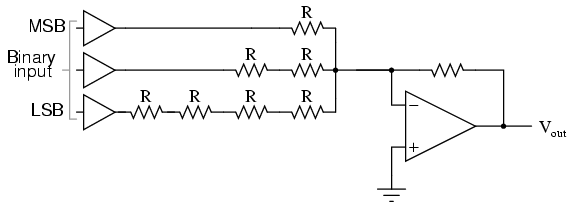Unfortunately, this approach merely substitutes one type of complexity for another: volume of components over diversity of component values. There is, however, a more efficient design methodology.

By constructing a different kind of resistor network on the input of our summing circuit, we can achieve the same kind of binary weighting with only two kinds of resistor values, and with only a modest increase in resistor count. This “ladder” network looks like this:Mathematically analyzing this ladder network is a bit more complex than for the previous circuit, where each input resistor provided an easily-calculated gain for that bit. For those who are interested in pursuing the intricacies of this circuit further, you may opt to use Thevenin’s theorem for each binary input (remember to consider the effects of the virtual ground), and/or use a simulation program like SPICE to determine circuit response. Either way, you should obtain the following table of figures:

———————————
| Binary | Output voltage |
———————————
| 000 | 0.00 V |
———————————
| 001 | -1.25 V |
———————————
| 010 | -2.50 V |
———————————
| 011 | -3.75 V |
———————————
| 100 | -5.00 V |
———————————
| 101 | -6.25 V |
———————————
| 110 | -7.50 V |
———————————
| 111 | -8.75 V |
———————————

As was the case with the binary-weighted DAC design, we can modify the value of the feedback resistor to obtain any “span” desired. For example, if we’re using +5 volts for a “high” voltage level and 0 volts for a “low” voltage level, we can obtain an analog output directly corresponding to the binary input (011 = -3 volts, 101 = -5 volts, 111 = -7 volts, etc.) by using a feedback resistance with a value of 1.6R instead of 2R.

Related

COMMENT songs

1. dro4er

I want to quote your post in my blog. It can?
And you et an account on Twitter?